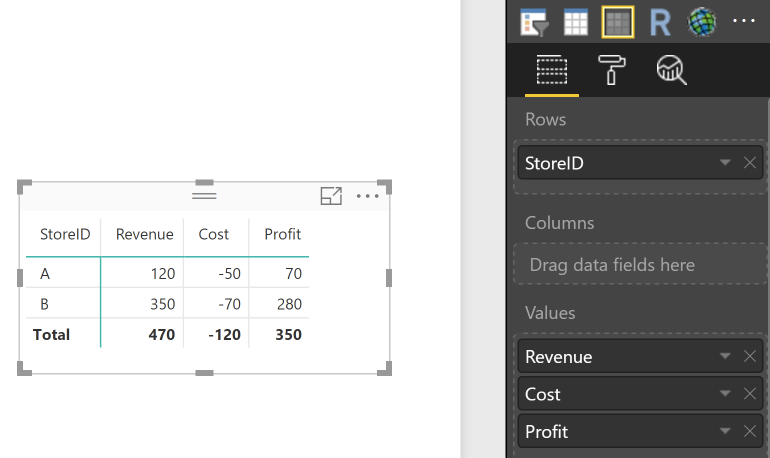2 views
in BI

How would I do a left join in DAX? When I try to adding a relationship or using the left outer join DAX function I get the following errors (see below). Any ideas would be greatly appreciated!

Error when creating relationship:Error when trying NaturalLeftOuterJoin()For reference, I'm trying to create calculated rows of an income statement.

Example:

• Revenue: 100
• Cost: 80
• Profit: 20 (Revenue-Cost)

My tables are like below:In SQL this is super easy - I just do a left outer join on the AccountID field which creates records for the Profit line, like below:

SELECT f.[YearMonth] ,f.[StoreID] ,f.[AccountID] ,f.[Amount] ,t.[TemplateID] ,t.[AccountID] ,t.[Line] FROM [dbo].[Fact] f left join [dbo].[Templates] t on f.[AccountID] = t.[AccountID]

Result:Then I can pivot it like so:In DAX it seems much more complex - hopefully, someone can prove me wrong on this! I've read bi-directional filtering may allow many-to-many relationships but I wasn't able to get it working here. The reason I'm trying to do this join in DAX rather than SQL because I have several statement templates and would prefer not having several loads with very similar data if it could be done dynamically through DAX. Thanks!

by (47.2k points)
• You can simply write a few Measures in DAX to do the calculations in Power BI (which is exactly the power of Power BI), and only the Fact table is needed.

• DAX:

Revenue:

Revenue = CALCULATE( SUM('Fact'[Amount]), FILTER( 'Fact', 'Fact'[Amount] > 0 ) )

Cost:

Cost = CALCULATE( SUM('Fact'[Amount]), FILTER( 'Fact', 'Fact'[Amount] < 0 ) )

Profit:

Profit = [Revenue] + [Cost]

Then you can use a Matrix visualization to get the desired results:• If you really need the Revenue/Cost/Profit to be in the row instead of column, you may need to pivot the data or write the calculations as new Column (but not Measure) instead. This is due to a product limitation in Power BI.